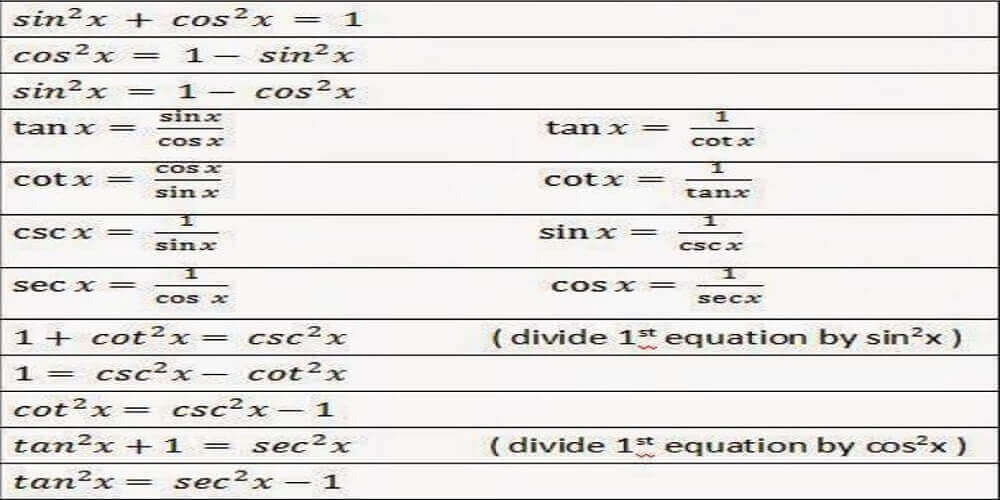IB Maths Tutors say that there are a lot of relationships amongst trigonometric ratios like Sin, Cos, Tan etc. There are many relationships that are true for all values of the variables. We call these relationships trigonometric identities. Our IB Tutors can give you a deep understanding of Trigonometric Identities
If we make a point P (x,y) on the unit circle making an anglewith the origin then

x=Sinand y=Cossquaring and adding both these values we get

x²+y²=Sin²θ+Cos²θ  on a unit circle   so                                  Sin²θ+Cos²θ=1   (i)

we can also write   Sin²θ=1-Cos²θ and

Cos²θ=1-Sin²θ

these are most widely used identities. If we divide equation (i) by Sin and Cos respectively then we get

Cosec²θ=1+Cot²θ and

Sec²θ=1+Tan²θ

We can also write these relationships by shifting Cot and Tan and thus we get more Trigonometric identities.
So we see that equation (i) in itself is a creator of nine identities in total## How to Solve Questions Based on Trigonometric Identities-

Although every trigonometric identities problem is unique in its own way yet we can apply a few simple steps to solve the majority of them

►First of all, we must check for the common value in the question

► Check if any direct Trigonometric identity is given in the question or not, if yes then we should apply it

► Convert every given trigonometric ratio in Sin/Cos

►Take L.C.M

► Simplify everything to get the required result

For simplification, we can use algebraic identities like- (a+b)², (a+b)³, a²-b², and a³+b³ etc.

### Let us now try some questions based on the above tricks

Question-1 Prove That

(cosec θ – cot θ)= (1-cos θ)/(1+cos θ)

Solution:

L.H.S

(Cosecθ-Cotθ)²=(1/Sinθ-Cosθ/Sinθ)²

=(1-Cosθ/Sinθ)²
=(1-Cosθ)²/Sin²θ
=(1-Cosθ)²/1-Cos²θ
=(1-Cosθ)²/(1-Cosθ)(1+Cosθ)
=(1-Cosθ)/(1+Cosθ)
Question-2   Show that
cos A/(1+sin A) + (1+sin A)/cos A = 2 sec A
Solution:
L.H.S. cos A/(1+sin A) + (1+sin A)/cos A
= [cos2A +(1+sinA)2]/(1+sin A)cos A
= (cos2A + sin2A + 1 + 2sin A)/(1+sin A)cos A
= (1 + 1 + 2sin A)/(1+sin A)cos A
= (2+ 2sin A)/(1+sin A)cos A
= 2(1+sin A)/(1+sin A)cos A
= 2/cos A = 2 sec A = R.H.S.
Question- 3

If acosθ – bsinθ = c, prove that

asinθ + bcosθ = ±√a²+b²-c²

Solution:

(acosθ – bsinθ)² + (asinθ + bcosθ)²

= (a²cos²θ + b²sin²θ – 2ab sinθcosθ)+ (a²sin²θ + b²cos²θ + 2ab sinθcosθ)

= (a²cos²θ + b²sin²θ ) + (a²sin²θ + b²cos²θ)

= a²(cos²θ +sin²θ) + b²(cos²θ +sin²θ)

=a²+b²

####Trigonometric identities Worksheet.pdf

You can read and share my other posts on Trigonometry

#### Third post -Concept three

Trigonometric Identity is a huge topic in IB Mathematics. It contains many formulas like double angle formula, compound angle formula, Sine Rule, Cosine Rule etc so I will keep on posting on this topic